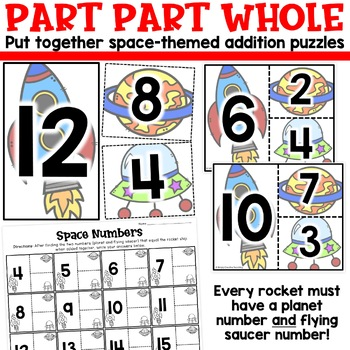Total:
\$0.00# 1st Grade Finding Unknown Numbers & Missing Addend Math Centers for 1.OA.81st
Subjects
Standards
Resource Type
Formats Included
• PDF
Pages
27 pages

#### Also included in

1. These are 1st grade math centers for EVERY 1st Grade Common Core standard. For every 1st grade math standard, I'vecraeted 3-5 math centers or math stations that your students will love!2nd Grade Math Centers: Find them for every standard here!These 1st grade math centers include board games, task ca
\$65.00
\$84.00
Save \$19.00
2. This is a MINI BUNDLE of my 1st grade addition and subtraction math centers for Operations & Algebraic Thinking Standards (1.OA.1, 1.OA.2, 1.OA.3, 1.OA.4, 1.OA.5, 1.OA.6, 1.OA.7, & 1.OA.8). For each 1st grade OA standard, I've created 3-5 math centers/stations that your students will love!Th
\$26.00
\$32.00
Save \$6.00

### Description

Practice first grade unknown numbers with these math centers! They align with 1st grade Common Core standard 1.OA.8 for students to work on determining the unknown missing number or addend in addition and subtraction using part part whole. They consist of puzzles, cards to solve, matching activities, and more! All equations & unknown numbers are within 20. They are designed for you to use in your math centers and rotations.

Included are 4 different math stations for 1st grade unknown numbers:

1. Space Numbers: Students will use part-part-whole to put together addition puzzles.
2. Monkey Missing Numbers: Students will match/solve word problems with missing numbers to the correct equation.
3. Movie Missing Numbers: Students will choose a card and determine the missing number in the equation.
4. Missing Number Puzzles: Students will find the four equations that have the same missing number for each puzzle.

Total Pages
27 pages
N/A
Teaching Duration
N/A
Report this Resource to TpT
Reported resources will be reviewed by our team. Report this resource to let us know if this resource violates TpT’s content guidelines.

### Standards

to see state-specific standards (only available in the US).
Determine the unknown whole number in an addition or subtraction equation relating three whole numbers. For example, determine the unknown number that makes the equation true in each of the equations 8 + ? = 11, 5 = ▯ - 3, 6 + 6 = ▯.